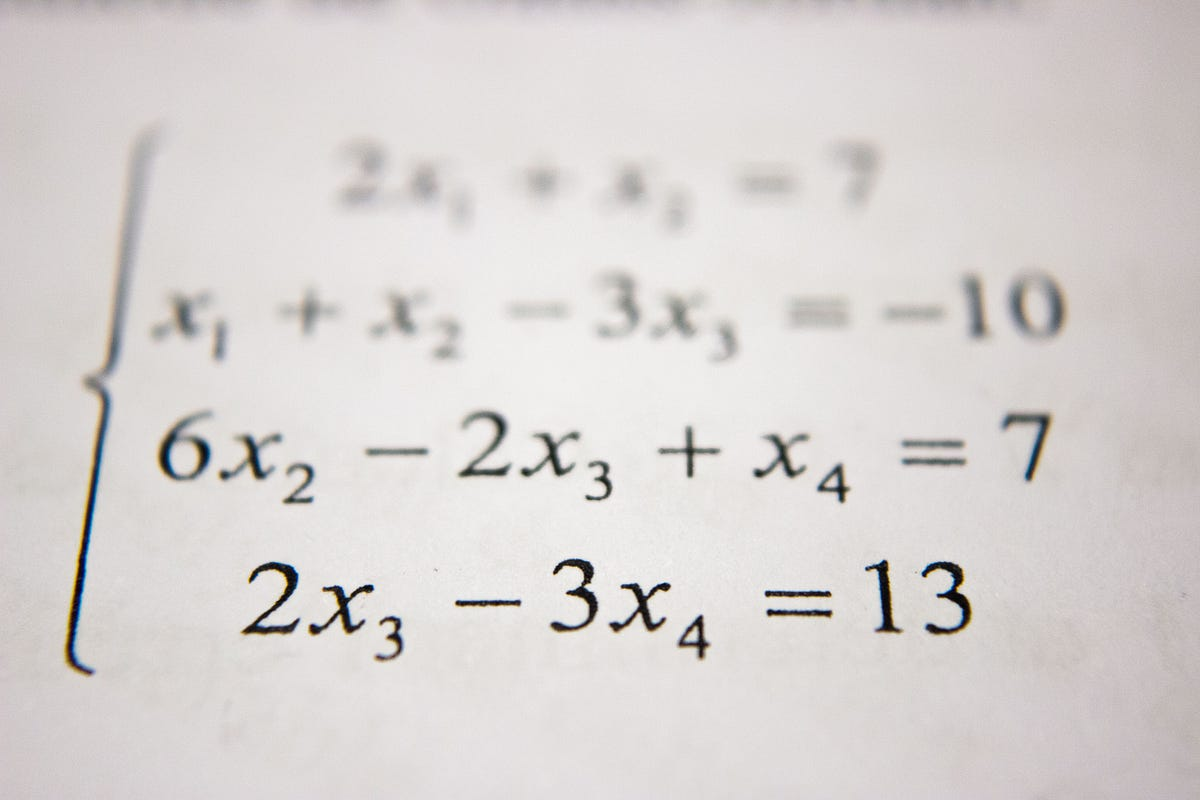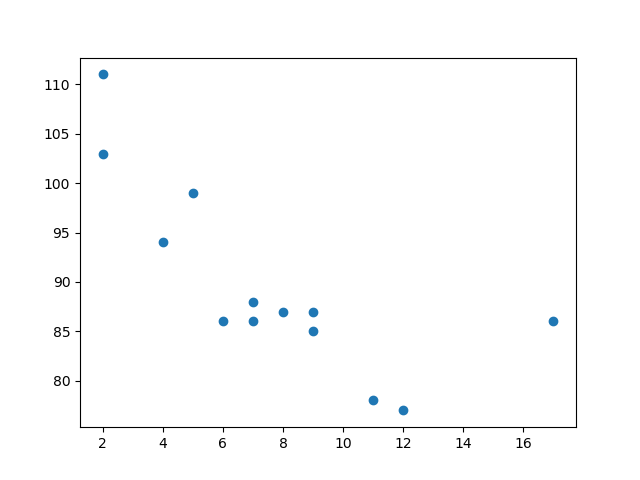# The Ultimate Beginners Guide to Linear Regression in Python.The term regression is used when you try to find the relationship between variables. Machine Learning is making the computer learn from studying data and statistics.

Machine Learning is making the computer learn from studying data and statistics. Machine Learning is a step into the direction of artificial intelligence (AI). Machine Learning is a program that analyses data and learns to predict the outcome.

### What is Regression?

The term regression is used when you try to find the relationship between variables. In Machine Learning and statistical modelling, that relationship is used to predict the outcome of future events.

Linear Regression

Linear regression uses the relationship between the data-points to draw a straight line through all them. This line can be used to predict future values.

### Getting Started

Python has methods for finding a relationship between data-points and to draw a line of linear regression. We will show you how to use these methods instead of going through the mathematic formula.

An example:

``````x = [5,7,8,7,2,17,2,9,4,11,12,9,6]
y = [99,86,87,88,111,86,103,87,94,78,77,85,86]

plt.scatter(x, y)
plt.show()``````

This displays a scatter plot:Import ‘scipy’ and draw the line of Linear Regression:

``````import matplotlib.pyplot as plt
from scipy import stats``````

Create the arrays that represent the values of the x and y-axis:

``````x = [5,7,8,7,2,17,2,9,4,11,12,9,6]
y = [99,86,87,88,111,86,103,87,94,78,77,85,86]``````

## AI(Artificial Intelligence): The Business Benefits of Machine Learning

Enroll now at CETPA, the best Institute in India for Artificial Intelligence Online Training Course and Certification for students & working professionals & avail 50% instant discount.

## Pipelines in Machine Learning | Data Science | Machine Learning | Python

Machine Learning Pipelines performs a complete workflow with an ordered sequence of the process involved in a Machine Learning task. The Pipelines can also

## Learning in Artificial Intelligence - Great Learning

What is Artificial Intelligence (AI)? AI is the ability of a machine to think like human, learn and perform tasks like a human. Know the future of AI, Examples of AI and who provides the course of Artificial Intelligence?

## AutoML: Automated Machine Learning | Data Science | Machine Learning | Python

AutoML makes the power of a Machine Learning algorithm available to you even if you don't have the complete knowledge of Machine Learning.You can use AutoML

## Contact Tracing with Machine Learning | Data Science | Machine Learning | Python

I will be proposing a contact tracing algorithm that relies on GPS data, which can be used in contact tracing with machine learning.